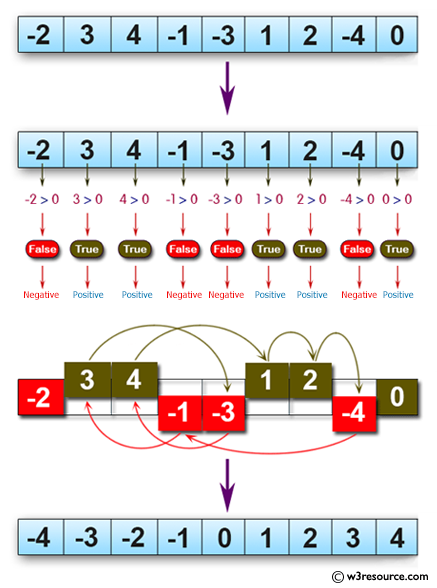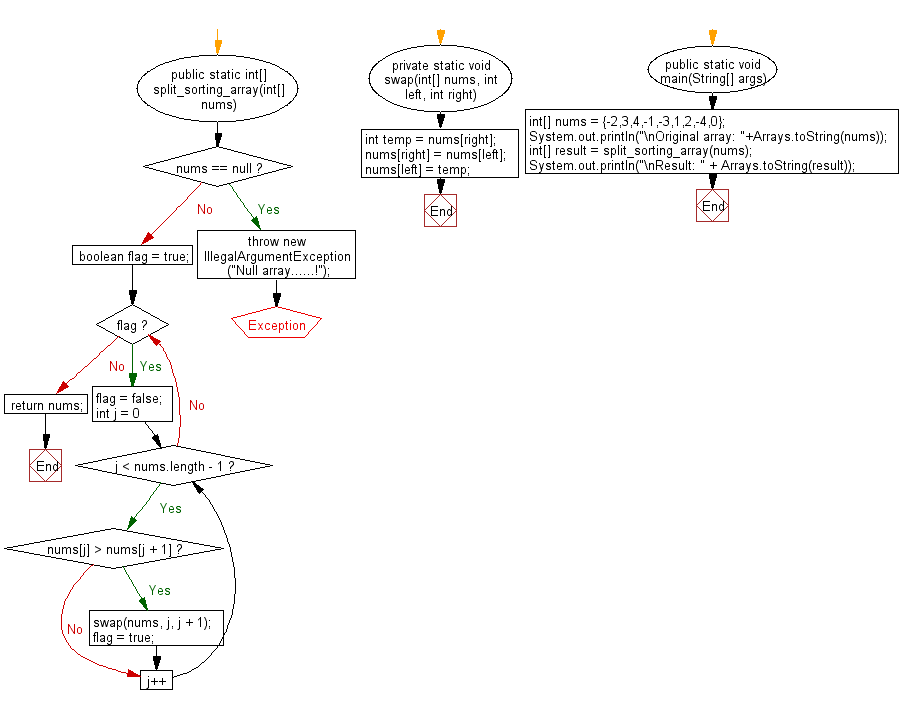﻿ Java programming exercises: Move every positive number to the right and every negative number to the left of a given array of integers - w3resource# Java Exercises: Move every positive number to the right and every negative number to the left of a given array of integers

## Java Basic: Exercise-165 with Solution

Write a Java program to move every positive number to the right and every negative number to the left of a given array of integers.

Pictorial Presentation:Sample Solution:

Java Code:

``````import java.util.*;
public class Solution {
public static int[] split_sorting_array(int[] nums) {
if (nums == null) {
throw new IllegalArgumentException("Null array......!");
}
boolean flag = true;
while (flag) {
flag = false;
for (int j = 0; j < nums.length - 1; j++) {
if (nums[j] > nums[j + 1]) {
swap(nums, j, j + 1);
flag = true;
}
}
}
return nums;
}
private static void swap(int[] nums, int left, int right) {
int temp = nums[right];
nums[right] = nums[left];
nums[left] = temp;
}
public static void main(String[] args) {
int[] nums = {-2,3,4,-1,-3,1,2,-4,0};
System.out.println("\nOriginal array: " + Arrays.toString(nums));
int[] result = split_sorting_array(nums);
System.out.println("\nResult: " + Arrays.toString(result));
}
}
```
```

Sample Output:

```Original array: [-2, 3, 4, -1, -3, 1, 2, -4, 0]

Result: [-4, -3, -2, -1, 0, 1, 2, 3, 4]
```

Flowchart:Java Code Editor:

What is the difficulty level of this exercise?

﻿

## Java: Tips of the Day

Parsing dates:

```import java.io.*;
import java.util.*;
import java.text.*;

String s = "2001/09/23 14:39";

SimpleDateFormat formatter = new SimpleDateFormat ("yyyy/MM/dd H:mm");
Date d = formatter.parse(s, new ParsePosition(0));
```